﻿ 基于深度学习的天气雷达回波序列外推及效果分析
 气象学报2021, Vol. 79Issue (5): 817-827PDF
http://dx.doi.org/10.11676/qxxb2021.041

0

#### 文章信息

HUANG Xingyou, MA Yurong, HU Suman. 2021.

Extrapolation and effect analysis of weather radar echo sequence based on deep learning

Acta Meteorologica Sinica, 79(5): 817-827.
http://dx.doi.org/10.11676/qxxb2021.041

### 文章历史

2021-01-15 收稿
2021-04-30 改回

1. 南京信息工程大学气象灾害预报预警与评估协同创新中心，南京，210044;
2. 南京信息工程大学，南京，210044

Extrapolation and effect analysis of weather radar echo sequence based on deep learning
HUANG Xingyou1,2, MA Yurong2 , HU Suman2
1. Collaborative Innovation Center on Forecast and Evaluation of Meteorological Disasters，Nanjing University of Information Science and Technology，Nanjing 210044，China;
2. Nanjing University of Information Science and Technology，Nanjing 210044，China
Abstract: Weather radar data is the main reference for nowcasting of severe convective weather. To address the problems of insufficient data utilization and limited extrapolation time in the radar echo extrapolation method widely used in China, the neural network is applied to radar echo extrapolation. The predictive neural network model gives 2 h prediction results of radar echo variation. The essence of radar echo extrapolation problem is the spatiotemporal sequence prediction problem. The network has long and short time memory unit (Long Short-Term Memory, LSTM) to solve the time memory problem and convolutional layers to extract spatial features. The training and testing datasets are constructed using radar data from Fujian, Jiangsu and Henan for several years. Considering the fact that the frequencies of different rainfall levels are highly imbalanced, the network is trained by weighted loss function to improve the prediction accuracy of strong echoes. The test set and individual case evaluation show that CSI (Critical Succes Index) and POD (Probability Of Detection) of predictive neural network are higher than that of optical flow method and FAR (False Alarm Ratio) is lower than that of optical flow method under the same extrapolation aging and reflectivity threshold. Among different precipitation types, the SSIM (structural similarity) value of the predictive neural network is higher than that of the optical flow method, and the SSIM value of the stratiform-cloud precipitation is larger than that of the convective-cloud precipitation. Therefore, the predictive neural network has a better ability to predict strong echoes than optical flow. In terms of the timeliness of forecasting, the predictive neural network model has certain advantages, and it is more accurate in forecasting stratiform-cloud precipitation than forecasting convective-cloud precipitation.
Key words: Nowcasting    Recurrent neural network    Convolutional neural network    Loss function    Deep learning
1 引　言

2 方　法 2.1 循环神经网络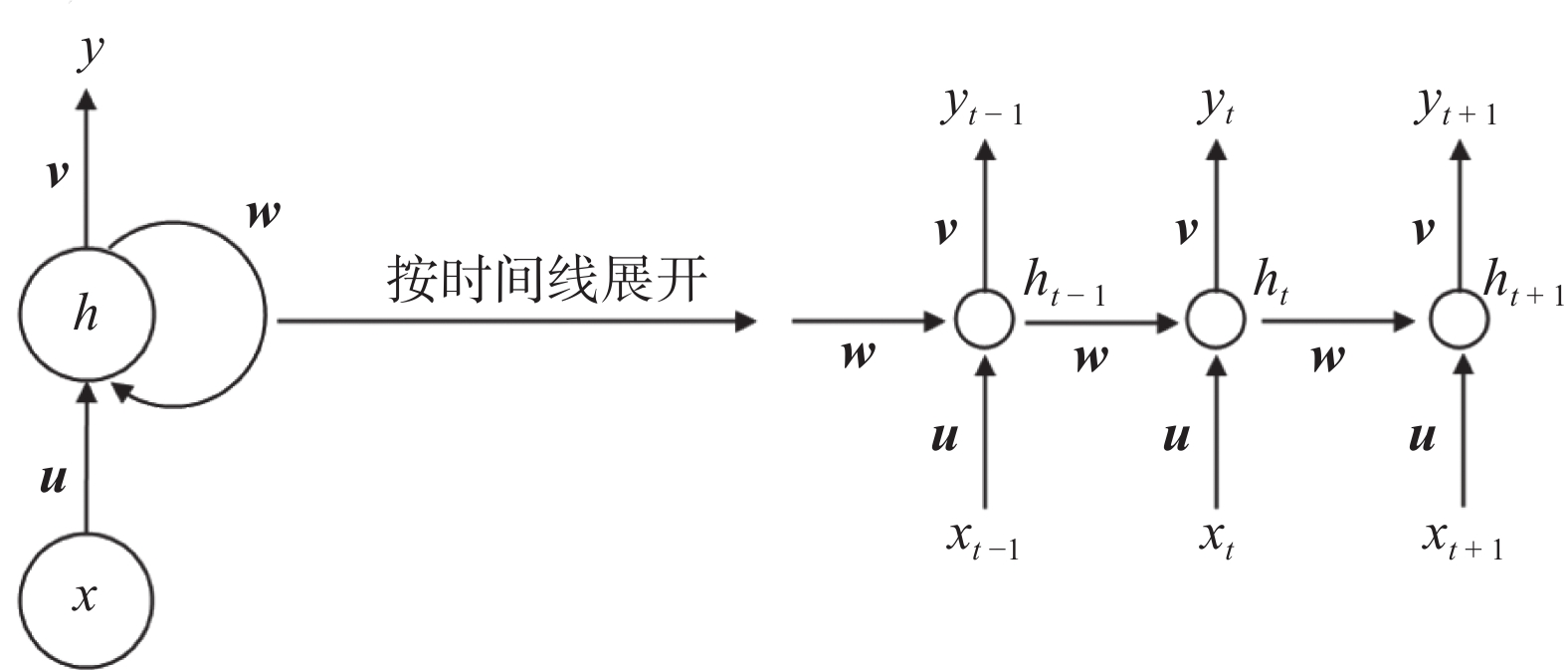图 1  循环神经网络 Fig. 1  Recurrent neural network

 ${h_t} = f({\boldsymbol u} {\text •} {x_t} + {\boldsymbol w} {\text •}{h_{t - 1}})$ (1)
 ${y_t} = g({\boldsymbol v} {\text •} {h_t})$ (2)

2.2 网络模型

2.3 损失函数

 $w(x) = \left\{ {\begin{array}{*{20}{l}} 1&{x \in [0,20]} \\ {10}&{x \in (20,30]} \\ {15}&{x \in (30,40]} \\ {30}&{x \in (40,50]} \\ {50}&{x \in (50,75]} \end{array}} \right.$ (3)

 ${{{E}}_{\rm{s}}} = \frac{\,1\,}{\,n\,}\sum\limits^{N}_{n=1} {\sum\limits_{i = 1}^{400} {\sum\limits_{j = 1}^{400} {{w_{n,i,j}}} } } {({x_{n,i,j}} - {\hat x{_{n,i,j}}})^2}$ (4)
 ${E_{\rm a}} = \frac{\,1\,}{\,n\,}\sum\limits_{n=1}^N {\sum\limits_{i = 1}^{400} {\sum\limits_{j = 1}^{400} {{w_{n,i,j}}} } } \left| {{x_{n,i,j}} - {{\hat x}{_{n,i,j}}}} \right|$ (5)

3 数据集构造 3.1 数据质量控制

3.2 雷达数据格点化

3.3 样本数据集的生成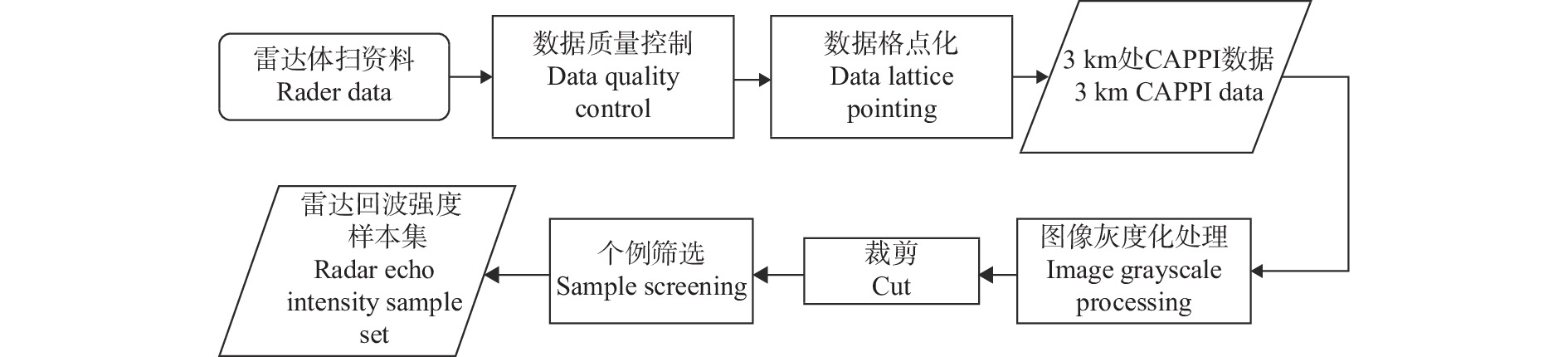图 2  数据集构造流程 Fig. 2  Process of constructing data sets图 3  数据集中一组样本可视化后图像 Fig. 3  A group of visualized sample images in the dataset
4 试验结果与分析 4.1 模型评价指标

 $\begin{split}\\ \\{\rm{CSI}} = \frac{a}{{a + b + c}}\end{split}$ (6)
 ${\rm{POD}} = \frac{a}{{a + c}}$ (7)
 ${\rm{FAR}} = \frac{b}{{a + b}}$ (8)

 ${\rm{SSIM}}(x,y) = \frac{{(2{\mu _x}{\mu _y} + {C_1})(2{\sigma _{xy}} + {C_2})}}{{(\mu _x^2 + \mu _y^2 + {C_1})(\sigma _x^2 + \sigma _y^2 + {C_2})}}$ (9)

4.2 测试集检验

 评估指标 临界成功指数 命中率 虚警率 模型 光流法 预测神经网络 光流法 预测神经网络 光流法 预测神经网络 30 min 20 dBz 0.524 0.678 0.665 0.791 0.307 0.174 30 dBz 0.369 0.534 0.519 0.678 0.451 0.285 40 dBz 0.172 0.255 0.277 0.322 0.693 0.449 50 dBz 0.022 0.156 0.038 0.255 0.936 0.713 60 min 20 dBz 0.404 0.588 0.551 0.713 0.423 0.229 30 dBz 0.246 0.394 0.379 0.572 0.604 0.441 40 dBz 0.075 0.134 0.136 0.262 0.855 0.785 50 dBz 0.005 0.052 0.011 0.161 0.983 0.828 90 min 20 dBz 0.329 0.513 0.472 0.667 0.504 0.310 30 dBz 0.181 0.301 0.296 0.548 0.691 0.599 40 dBz 0.042 0.056 0.082 0.093 0.915 0.876 50 dBz 0.002 0.018 0.005 0.026 0.992 0.944 120 min 20 dBz 0.272 0.475 0.311 0.582 0.569 0.346 30 dBz 0.140 0.231 0.244 0.393 0.749 0.641 40 dBz 0.027 0.042 0.058 0.072 0.943 0.908 50 dBz 0.001 0.006 0.003 0.011 0.996 0.986
4.3 个例分析 4.3.1 层状云降水过程1图 4  2017年5月3日层状云降水地面实况与模型预报结果 Fig. 4  Comparison between ground truth and forecast on 3 May 2017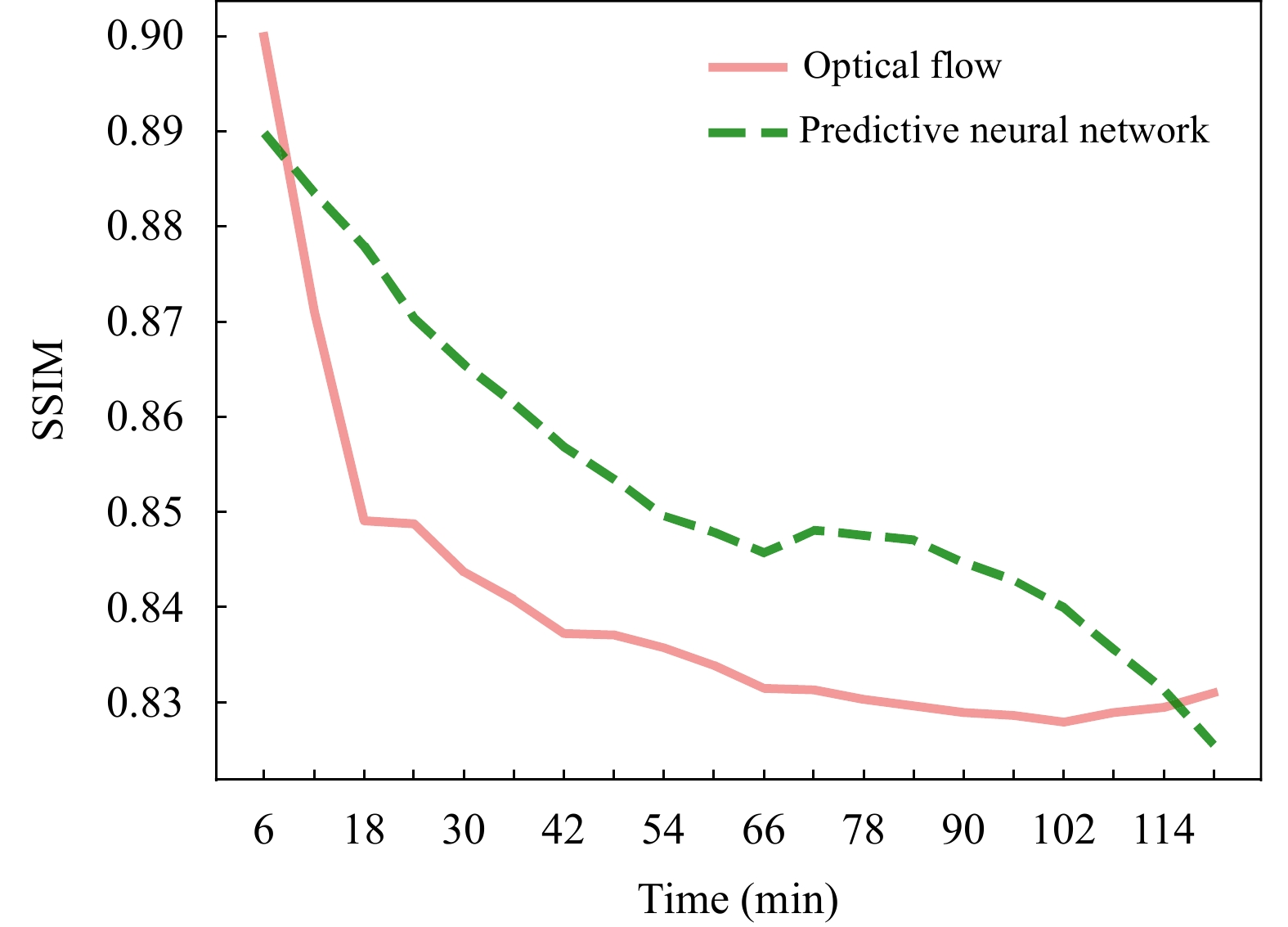图 5  模型预报结果与地面实况的SSIM Fig. 5  SSIM of model predicted results and ground truth
4.3.2 层状云降水过程2图 6  2018年6月18日层状云降水地面实况与模型预报结果 Fig. 6  Comparison between ground truth and forecast on 18 June 2018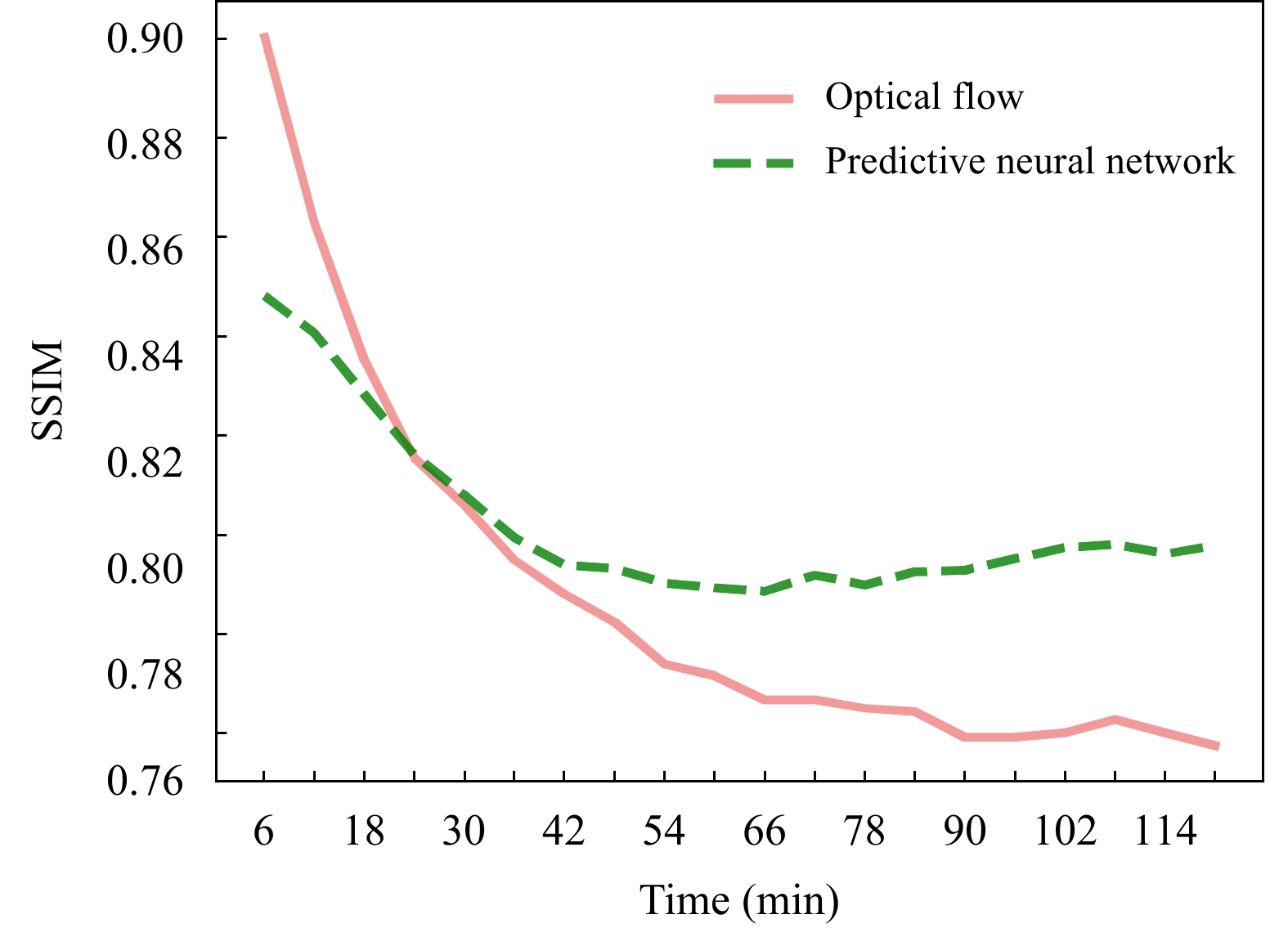图 7  模型预报结果与地面实况的SSIM Fig. 7  SSIM of model predicted results and ground truth
4.3.3 对流云降水过程1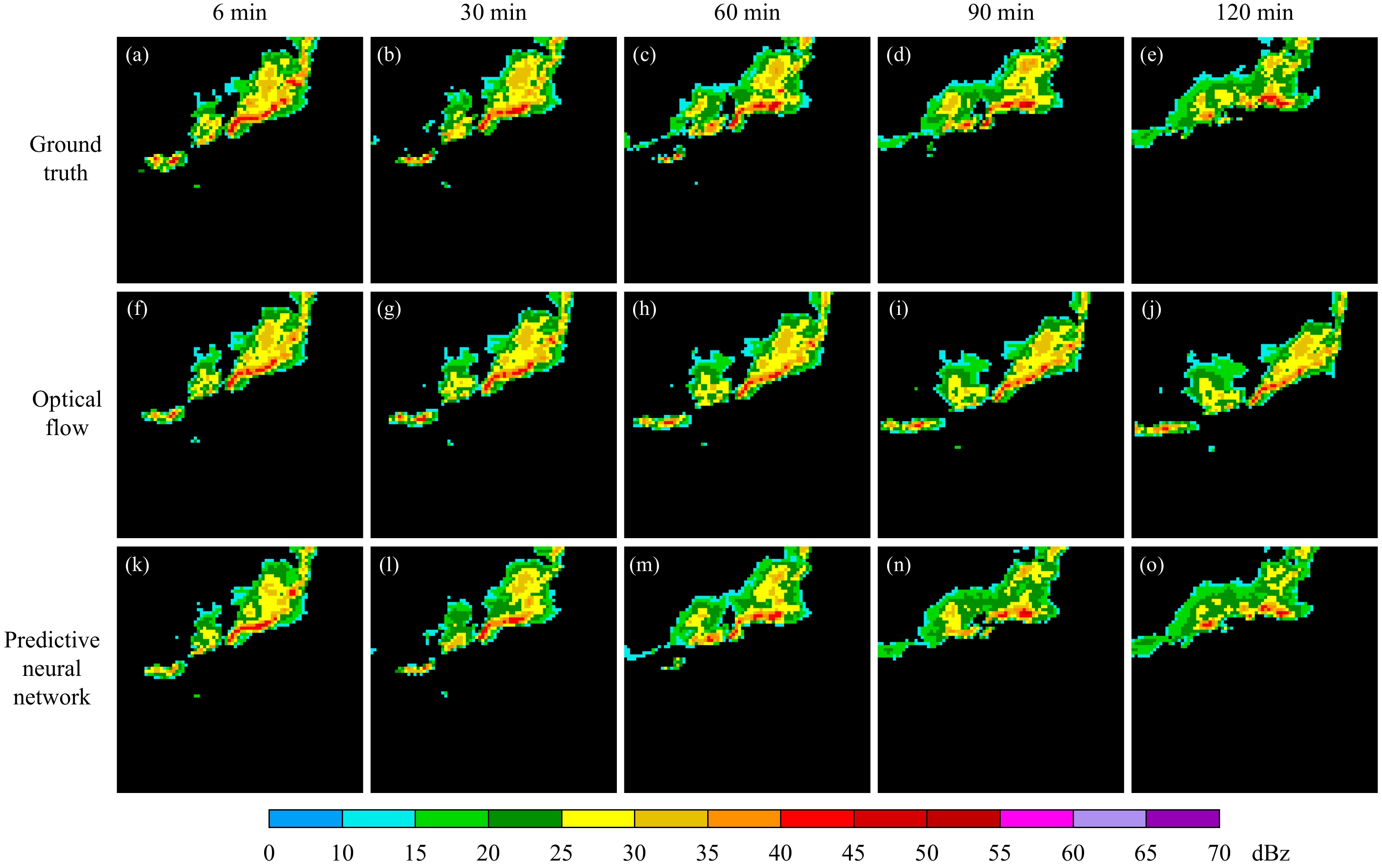图 8  2020年6月24日对流云降水地面实况与模型预报结果 Fig. 8  Comparison between ground truth and forecast on 24 June 2020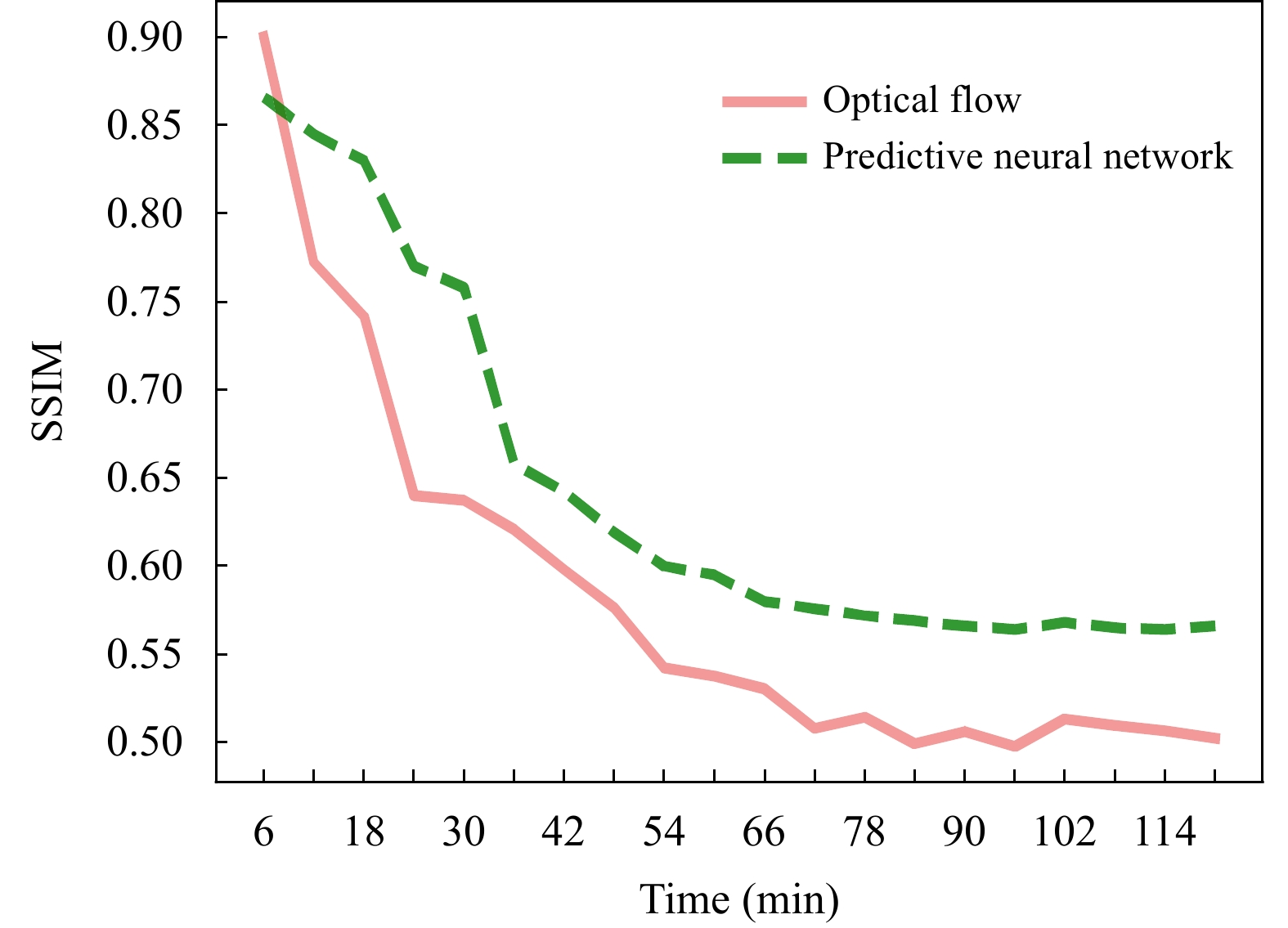图 9  模型预报结果与地面实况的SSIM Fig. 9  SSIM of model predicted results and ground truth
4.3.4 对流云降水过程2图 10  2018年8月18日对流云降水地面实况与模型预报结果 Fig. 10  Comparison between ground truth and forecast on 18 August 2018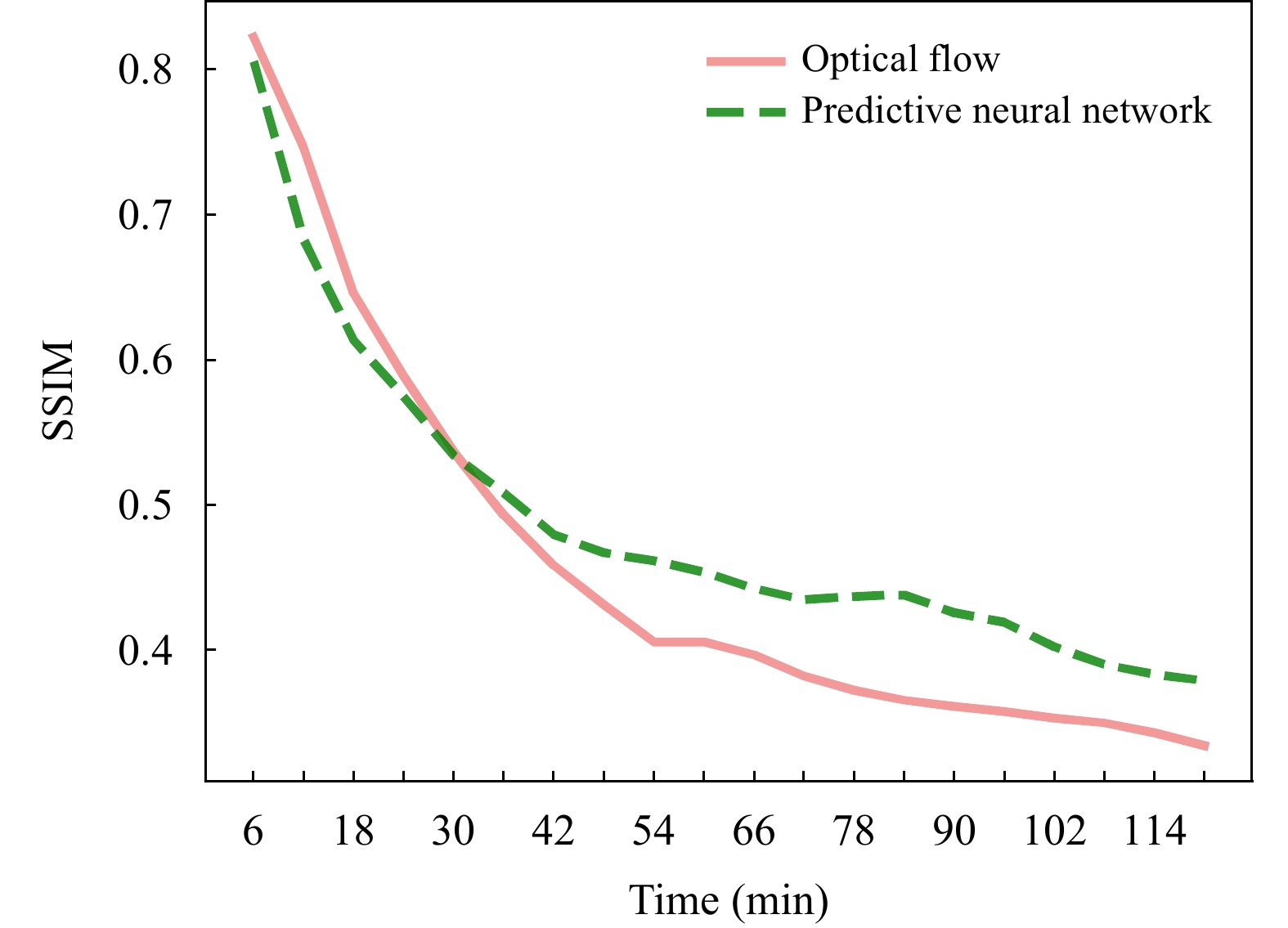图 11  模型预报结果与地面实况的SSIM Fig. 11  SSIM of model predicted results and ground truth
5 总结与讨论

（1）雷达回波外推的本质是时、空序列的预测问题，神经网络具有较强的非线性映射能力，可以充分利用大量的历史数据对雷达回波进行非线性外推。文中使用的预测网络模型具有提取空间特征的卷积模块和解决时间记忆问题的改进后的LSTM结构单元。结果表明预测神经网络对雷达回波外推的效果优于光流法。

（2）考虑到不同降水强度出现的频率不同，强降水对人类活动影响较大，网络在训练过程中赋予强回波较大的权重以提高对强回波的预报能力。测试集检验结果表明，预测神经网络2 h的回波外推在40和50 dBz检验阈值项上，临界成功指数、命中率较光流法均有所提高，虚警率有所下降，表明该网络对强回波的预报能力高于光流法。

（3）4个降水过程个例表明，光流法和预测神经网络对层状云降水过程的预报准确率均高于对流云降水，主要是由于层状云降水回波变化缓慢，对流云降水强回波变化较快。尽管两种临近预报方案的预报效果随预报时间的延长而下降，但是预测神经网络比光流法仍具有优越性。

（4）基于深度学习外推的4个降水个例的预测雷达回波在后期出现模糊现象，可能的原因是雷达回波的预测具有不确定性，随着时间的推移，对细节的预测难度更大，因此网络会更注重回波整体形态上的演变，而丢失了细节性的纹理。